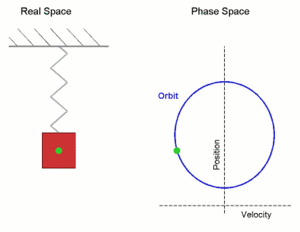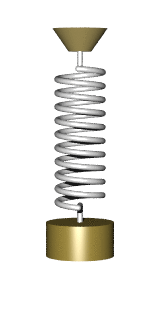# Simple harmonic motion

In mechanics and physics, simple harmonic motion is a type of periodic motion or oscillation motion where the restoring force is directly proportional to the displacement and acts in the direction opposite to that of displacement .

Simple harmonic motion can serve as a mathematical model for a variety of motions, such as the oscillation of a spring. In addition, other phenomena can be approximated by simple harmonic motion, including the motion of a simple pendulum as well as molecular vibration. Simple harmonic motion is typified by the motion of a mass on a spring when it is subject to the linear elastic restoring force given by Hooke's Law. The motion is sinusoidal in time and demonstrates a single resonant frequency. For simple harmonic motion to be an accurate model for a pendulum, the net force on the object at the end of the pendulum must be proportional to the displacement. This will be a good approximation when the angle of swing is small.

Simple harmonic motion provides a basis for the characterization of more complicated motions through the techniques of Fourier analysis.

## Introduction

The motion of a particle moving along a straight line with an acceleration which is always towards a fixed point on the line and whose magnitude is proportional to the distance from the fixed point is called simple harmonic motion [SHM].Simple harmonic motion shown both in real space and phase space. The orbit is periodic. (Here the velocity and position axes have been reversed from the standard convention to align the two diagrams)

In the diagram, a simple harmonic oscillator, consisting of a weight attached to one end of a spring, is shown. The other end of the spring is connected to a rigid support such as a wall. If the system is left at rest at the equilibrium position then there is no net force acting on the mass. However, if the mass is displaced from the equilibrium position, the spring exerts a restoring elastic force that obeys Hooke's law.

Mathematically, the restoring force F is given bywhere F is the restoring elastic force exerted by the spring (in SI units: N), k is the spring constant (N·m−1), and x is the displacement from the equilibrium position (m).

For any simple mechanical harmonic oscillator:

• When the system is displaced from its equilibrium position, a restoring force that obeys Hooke's law tends to restore the system to equilibrium.

Once the mass is displaced from its equilibrium position, it experiences a net restoring force. As a result, it accelerates and starts going back to the equilibrium position. When the mass moves closer to the equilibrium position, the restoring force decreases. At the equilibrium position, the net restoring force vanishes. However, at x = 0, the mass has momentum because of the acceleration that the restoring force has imparted. Therefore, the mass continues past the equilibrium position, compressing the spring. A net restoring force then slows it down until its velocity reaches zero, whereupon it is accelerated back to the equilibrium position again.

As long as the system has no energy loss, the mass continues to oscillate. Thus simple harmonic motion is a type of periodic motion.

## Dynamics of simple harmonic motion

For one-dimensional simple harmonic motion, the equation of motion, which is a second-order linear ordinary differential equation with constant coefficients, can be obtained by means of Newton's second law (and Hooke's law for a mass on a spring).where m is the inertial mass of the oscillating body, x is its displacement from the equilibrium (or mean) position, and k is a constant (the spring constant for a mass on a spring).

Therefore,Solving the differential equation above produces a solution that is a sinusoidal function.This equation can be written in the form:whereIn the solution, c1 and c2 are two constants determined by the initial conditions, and the origin is set to be the equilibrium position.[A] Each of these constants carries a physical meaning of the motion: A is the amplitude (maximum displacement from the equilibrium position), ω = 2πf is the angular frequency, and φ is the phase.[B]

Using the techniques of calculus, the velocity and acceleration as a function of time can be found:Speed:Maximum speed: ωA (at equilibrium point)Maximum acceleration: 2 (at extreme points)

By definition, if a mass m is under SHM its acceleration is directly proportional to displacement.whereSince ω = 2πf,and, since T = 1/f where T is the time period,These equations demonstrate that the simple harmonic motion is isochronous (the period and frequency are independent of the amplitude and the initial phase of the motion).

## Energy

Substituting ω2 with k/m, the kinetic energy K of the system at time t isand the potential energy isThe total mechanical energy has a constant value## ExamplesThe following physical systems are some examples of simple harmonic oscillator.

### Mass on a spring

A mass m attached to a spring of spring constant k exhibits simple harmonic motion in closed space. The equationshows that the period of oscillation is independent of both the amplitude and gravitational acceleration. The above equation is also valid in the case when an additional constant force is being applied on the mass, i.e. the additional constant force cannot change the period of oscillation.

### Uniform circular motion

Simple harmonic motion can be considered the one-dimensional projection of uniform circular motion. If an object moves with angular speed ω around a circle of radius r centered at the origin of the xy-plane, then its motion along each coordinate is simple harmonic motion with amplitude r and angular frequency ω.

### Mass of a simple pendulumIn the small-angle approximation, the motion of a simple pendulum is approximated by simple harmonic motion. The period of a mass attached to a pendulum of length l with gravitational acceleration g is given byThis shows that the period of oscillation is independent of the amplitude and mass of the pendulum but not of the acceleration due to gravity (g), therefore a pendulum of the same length on the Moon would swing more slowly due to the Moon's lower gravitational field strength.

This approximation is accurate only for small angles because of the expression for angular acceleration α being proportional to the sine of the displacement angle:where I is the moment of inertia. When θ is small, sin θθ and therefore the expression becomeswhich makes angular acceleration directly proportional to θ, satisfying the definition of simple harmonic motion.

### Scotch yoke

Main article: Scotch yoke

A Scotch yoke mechanism can be used to convert between rotational motion and linear reciprocating motion. The linear motion can take various forms depending on the shape of the slot, but the basic yoke with a constant rotation speed produces a linear motion that is simple harmonic in form.

## Notes

1. ^ The choice of using a cosine in this equation is arbitrary. Other valid formulations are:wheresince cos θ = sin(π/2θ).
2. ^ The maximum displacement (that is, the amplitude), xmax, occurs when cos(ωt ± φ) = 1, and thus when xmax = A.

## References

• Walker, Jearl (2011). Principles of Physics (9th ed.). Hoboken, N.J. : Wiley. ISBN 0-470-56158-0.
• Thornton, Stephen T.; Marion, Jerry B. (2003). Classical Dynamics of Particles and Systems (5th ed.). Brooks Cole. ISBN 0-534-40896-6.
• John R Taylor (2005). Classical Mechanics. University Science Books. ISBN 1-891389-22-X.
• Grant R. Fowles; George L. Cassiday (2005). Analytical Mechanics (7th ed.). Thomson Brooks/Cole. ISBN 0-534-49492-7.